# NCERT Solutions Chapter 7 - Triangles (I), Class 9, Maths Notes | Study Additional Documents & Tests for Class 9 - Class 9

## Class 9: NCERT Solutions Chapter 7 - Triangles (I), Class 9, Maths Notes | Study Additional Documents & Tests for Class 9 - Class 9

The document NCERT Solutions Chapter 7 - Triangles (I), Class 9, Maths Notes | Study Additional Documents & Tests for Class 9 - Class 9 is a part of the Class 9 Course Additional Documents & Tests for Class 9.
All you need of Class 9 at this link: Class 9

Exercise 7.3

1. ΔABC and ΔDBC are two isosceles triangles on the same base BC and vertices A and D are on the same side of BC (see Fig. 7.39). If AD is extended to intersect BC at P, show that
(i) ΔABD ≅ ΔACD
(ii) ΔABP ≅ ΔACP
(iii) AP bisects ∠A as well as ∠D.
(iv) AP is the perpendicular bisector of BC.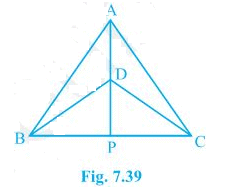Given,
ΔABC and ΔDBC are two isosceles triangles.

(i) In ΔABD and ΔACD,
AB = AC (ΔABC is isosceles)
BD = CD (ΔDBC is isosceles)
Therefore, ΔABD ≅ ΔACD by SSS congruence condition.

(ii) In ΔABP and ΔACP,
AP = AP (Common)
∠PAB = ∠PAC (ΔABD ≅ ΔACD so by CPCT)
AB = AC (ΔABC is isosceles)
Therefore, ΔABP ≅ ΔACP by SAS congruence condition.

(iii) ∠PAB = ∠PAC by CPCT as ΔABD ≅ ΔACD.
AP bisects ∠A. --- (i)
also,
In ΔBPD and ΔCPD,
PD = PD (Common)
BD = CD (ΔDBC is isosceles.)
BP = CP (ΔABP ≅ ΔACP so by CPCT.)
Therefore, ΔBPD ≅ ΔCPD by SSS congruence condition.
Thus, ∠BDP = ∠CDP by CPCT. --- (ii)
By (i) and (ii) we can say that AP bisects ∠A as well as ∠D.

(iv) ∠BPD = ∠CPD (by CPCT as ΔBPD ≅ ΔCPD)
and BP = CP --- (i)
also,
∠BPD + ∠CPD = 180° (BC is a straight line.)
⇒ 2∠BPD = 180°
⇒ ∠BPD = 90° ---(ii)
From (i) and (ii),
AP is the perpendicular bisector of BC.

2. AD is an altitude of an isosceles triangle ABC in which AB = AC. Show that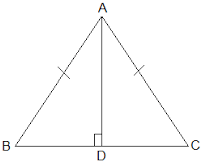Given,

AD is an altitude and AB = AC

(i) In ΔABD and ΔACD,

AB = AC (Given)
Therefore, ΔABD ≅ ΔACD by RHS congruence condition.
Now,
BD = CD (by CPCT)

3. Two sides AB and BC and median AM of one triangle ABC are respectively equal to sides PQ and QR and median PN of ΔPQR (see Fig. 7.40). Show that:
(i) ΔABM ≅ ΔPQN
(ii) ΔABC ≅ ΔPQR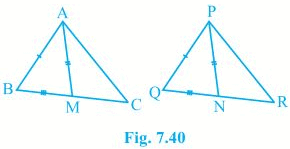Given,
AB = PQ, BC = QR and AM = PN

(i) 1/2 BC = BM and 1/2QR = QN (AM and PN are medians)
also,
BC = QR
⇒ 1/2 BC = 1/2QR
⇒ BM = QN
In ΔABM and ΔPQN,
AM = PN (Given)
AB = PQ (Given)
BM = QN (Proved above)
Therefore, ΔABM ≅ ΔPQN by SSS congruence condition.

(ii) In ΔABC and ΔPQR,
AB = PQ (Given)
∠ABC = ∠PQR (by CPCT)
BC = QR (Given)

Therefore, ΔABC ≅ ΔPQR by SAS congruence condition.

4. BE and CF are two equal altitudes of a triangle ABC. Using RHS congruence rule, prove that the triangle ABC is isosceles.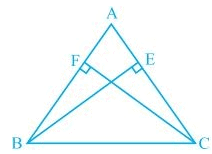Given,
BE and CF are two equal altitudes.
In ΔBEC and ΔCFB,
∠BEC = ∠CFB = 90° (Altitudes)
BC = CB (Common)
BE = CF (Common)
Therefore, ΔBEC ≅ ΔCFB by RHS congruence condition.
Now,
∠C = ∠B (by CPCT)
Thus, AB = AC as sides opposite to the equal angles are equal.

5. ABC is an isosceles triangle with AB = AC. Draw AP ⊥ BC to show that ∠B = ∠C.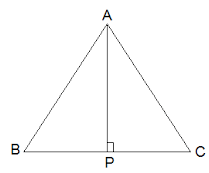Given,
AB = AC
In ΔABP and ΔACP,
∠APB = ∠APC = 90° (AP is altitude)
AB = AC (Given)
AP = AP (Common)
Therefore, ΔABP ≅ ΔACP by RHS congruence condition.
Thus, ∠B = ∠C (by CPCT)

Exercise 7.4

1. Show that in a right angled triangle, the hypotenuse is the longest side.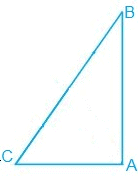ABC is a triangle right angled at B.
Now,
∠A + ∠B + ∠C = 180°
⇒ ∠A + ∠C = 90° and ∠B is 90°.
Since, B is the largest angle of the triangle, the side opposite to it must be the largest.
So, BC is the hypotenuse which is the largest side of the right angled triangle ABC.

2. In Fig. 7.48, sides AB and AC of ΔABC are extended to points P and Q respectively. Also, ∠PBC < ∠QCB. Show that AC > AB.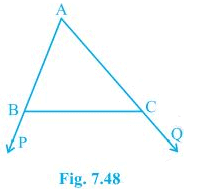Given,
∠PBC < ∠QCB
Now,
∠ABC + ∠PBC = 180°
⇒ ∠ABC = 180° - ∠PBC
also,
∠ACB + ∠QCB = 180°
⇒ ∠ACB = 180° - ∠QCB
Since,
∠PBC < ∠QCB therefore, ∠ABC > ∠ACB
Thus, AC > AB as sides opposite to the larger angle is larger.

3. In Fig. 7.49, ∠B < ∠A and ∠C < ∠D. Show that AD < BC.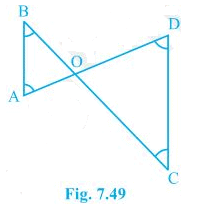Given,
∠B < ∠A and ∠C < ∠D
Now,
AO <  BO --- (i) (Side opposite to the smaller angle is smaller)
OD < OC ---(ii) (Side opposite to the smaller angle is smaller)
AO + OD < BO + OC

4. AB and CD are respectively the smallest and longest sides of a quadrilateral ABCD (see Fig. 7.50).
Show that ∠A > ∠C and ∠B > ∠D.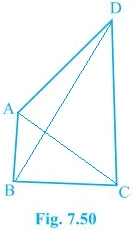In ΔABD,
∴ ∠ADB < ∠ABD --- (i) (Angle opposite to longer side is larger.)
Now,
In ΔBCD,
BC < DC < BD
∴ ∠BDC < ∠CBD --- (ii)
Adding (i) and (ii) we get,
∠ADB + ∠BDC < ∠ABD + ∠CBD
⇒ ∠B > ∠D
Similarly,
In ΔABC,
∠ACB < ∠BAC --- (iii) (Angle opposite to longer side is larger.)
Now,
∠DCA < ∠DAC --- (iv)
Adding (iii) and (iv) we get,
∠ACB + ∠DCA < ∠BAC + ∠DAC
⇒ ∠A > ∠C

5. In Fig 7.51, PR > PQ and PS bisects ∠QPR. Prove that ∠PSR > ∠PSQ.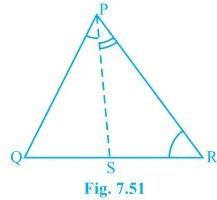Given,
PR > PQ and PS bisects ∠QPR
To prove,
∠PSR > ∠PSQ
Proof,
∠PQR > ∠PRQ --- (i) (PR > PQ as angle opposite to larger side is larger.)
∠QPS = ∠RPS --- (ii) (PS bisects ∠QPR)
∠PSR = ∠PQR + ∠QPS --- (iii) (exterior angle of a triangle equals to the sum of opposite interior angles)
∠PSQ = ∠PRQ + ∠RPS --- (iv) (exterior angle of a triangle equals to the sum of opposite interior angles)
∠PQR + ∠QPS > ∠PRQ + ∠RPS
⇒ ∠PSR > ∠PSQ [from (i), (ii), (iii) and (iv)]

6. Show that of all line segments drawn from a given point not on it, the perpendicular line segment is the shortest.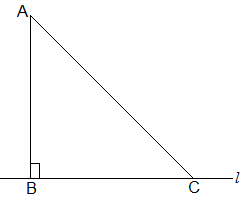Let l is a line segment and B is a point lying o it. We drew a line AB perpendicular to l. Let C be any other point on l.
To prove,
AB < AC
Proof,
In ΔABC,
∠B = 90°
Now,
∠A + ∠B + ∠C = 180°
⇒ ∠A + ∠C = 90°
∴ ∠C mustbe acute angle. or ∠C < ∠B
⇒ AB < AC (Side opposite to the larger angle is larger.)

The document NCERT Solutions Chapter 7 - Triangles (I), Class 9, Maths Notes | Study Additional Documents & Tests for Class 9 - Class 9 is a part of the Class 9 Course Additional Documents & Tests for Class 9.
All you need of Class 9 at this link: Class 9Use Code STAYHOME200 and get INR 200 additional OFF

## Additional Documents & Tests for Class 9

168 docs|11 tests

Track your progress, build streaks, highlight & save important lessons and more!

,

,

,

,

,

,

,

,

,

,

,

,

,

,

,

,

,

,

,

,

,

,

,

,

,

,

,

;